# Write balanced half-reactions for the following redox reaction: BiO3(aq)+6* (aq)+c202 (aq) — Bi3+(aq)+3H20(1)+2 CO2(aq) reduction: oxidation: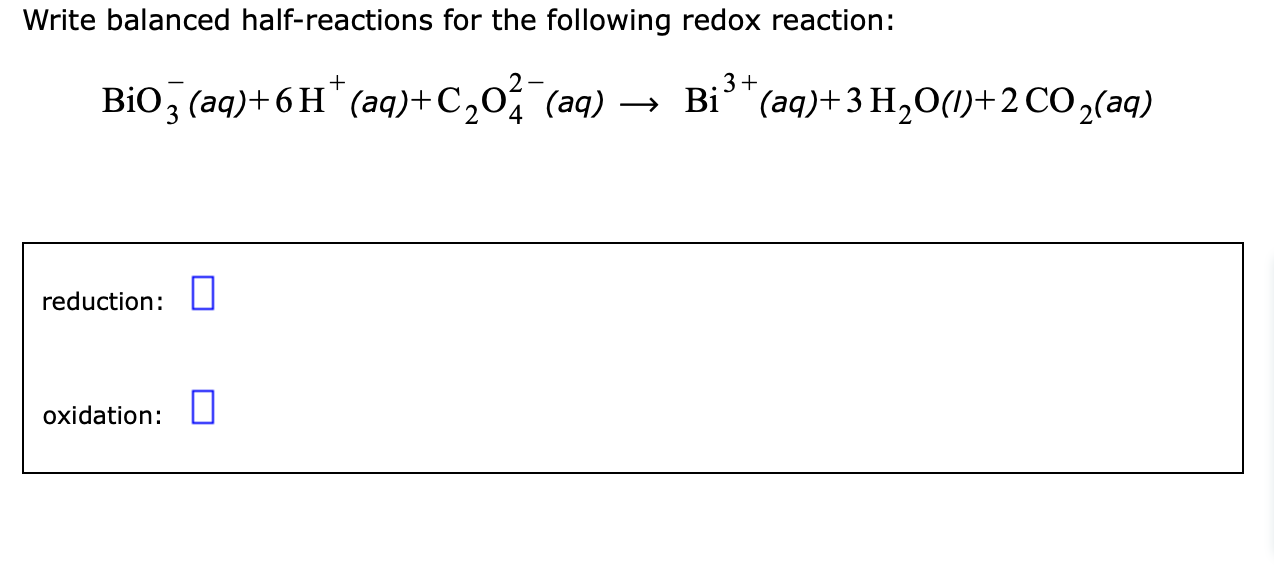Write balanced half-reactions for the following redox reaction: BiO3(aq)+6* (aq)+c202 (aq) — Bi3+(aq)+3H20(1)+2 CO2(aq) reduction: oxidation: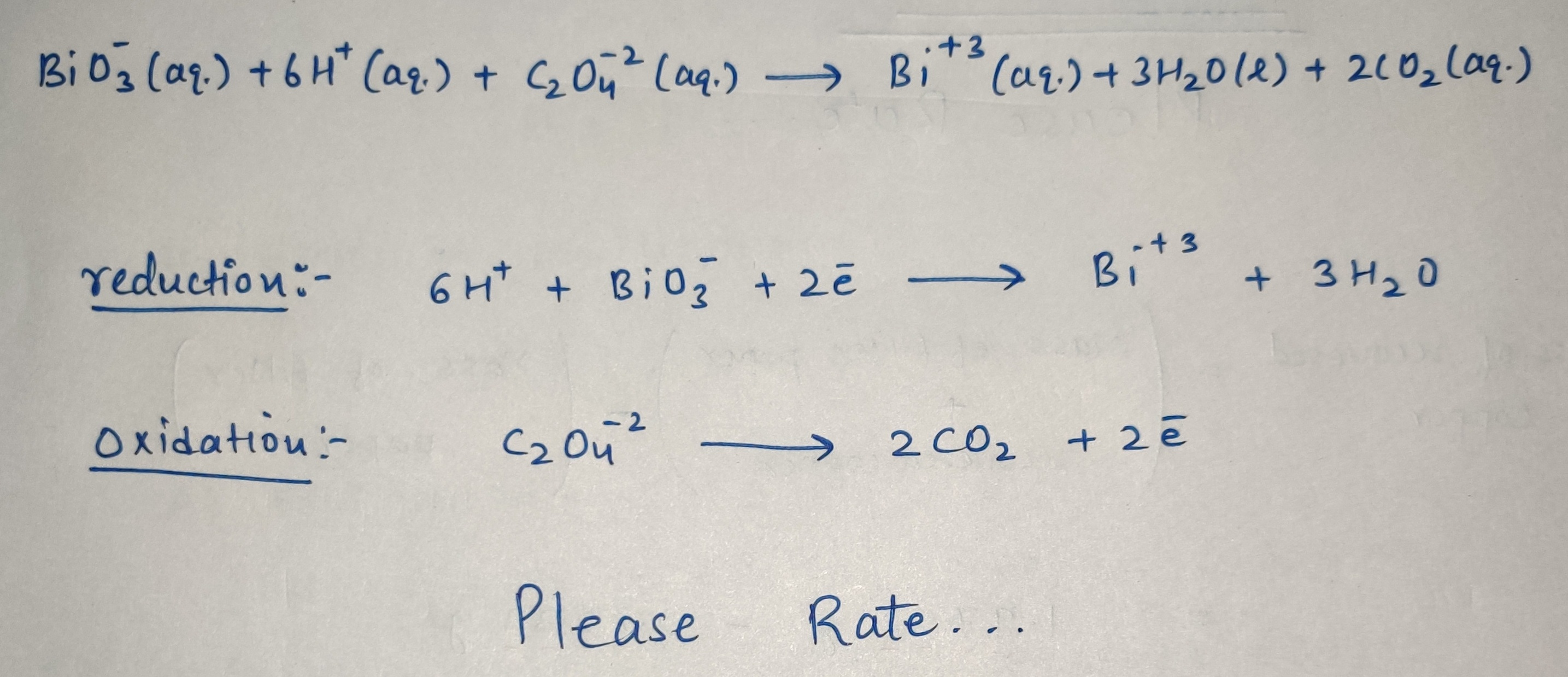#### Earn Coin

Coins can be redeemed for fabulous gifts.

Similar Homework Help Questions
• ### Write balanced half-reactions for the following redox reaction: reduction: 0 oxidation: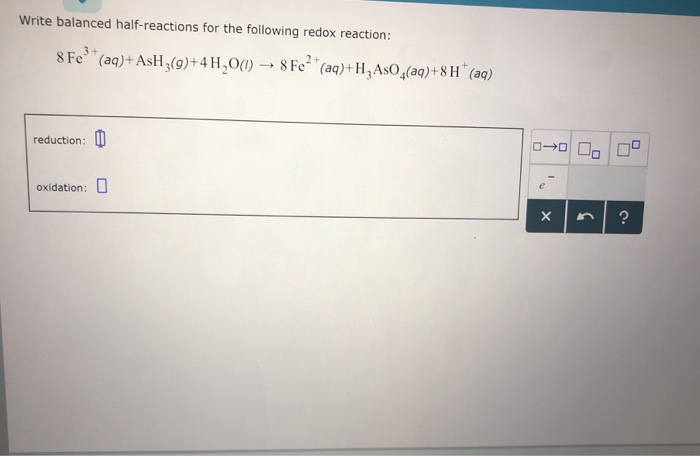Answer Write balanced half-reactions for the following redox reaction: reduction: 0 oxidation: Write balanced half-reactions for the following redox reaction: reduction: 0 oxidation:

• ### Write balanced half-reactions for the following redox reaction: Cr2O7^2-(aq)+14H^+(aq)+6Fe^+2(aq)? 2Cr^+3(aq)+7H2O(l)+6Fe^+3(aq) Reduction: Oxidation:

Write balanced half-reactions for the following redox reaction: Cr2O7^2-(aq)+14H^+(aq)+6Fe^+2(aq)? 2Cr^+3(aq)+7H2O(l)+6Fe^+3(aq) Reduction: Oxidation:

• ### Writing the wall reactions or a complex redox reaction in ... Write balanced half-reactions for the...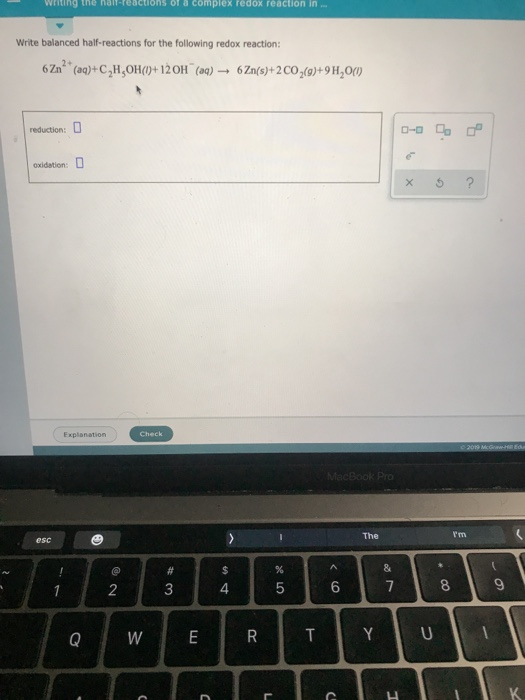Writing the wall reactions or a complex redox reaction in ... Write balanced half-reactions for the following redox reaction: 6 Zn* (aq)+C,H,OH(D+120H(aq) 6Zn(s)+2 CO2(9)+9H,000 reduction: 0 Oxidation: 0 x 5 ? Explanation Check MacBook Pro esc The I'm o WERTYuli lolle

• ### (1) Identify each of the following half-reactions as either an oxidation half-reaction or a reduction half-reaction....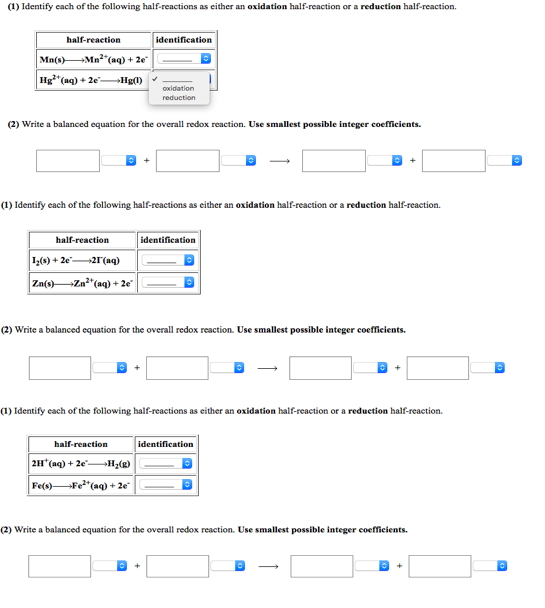(1) Identify each of the following half-reactions as either an oxidation half-reaction or a reduction half-reaction. half-reaction identification oxidation (2) Write a balanced equation for the overall redox reaction. Use smallest possible integer coefficients. (1) Identify each of the following half-reactions as either an oxidation half-reaction or a reduction half-reaction. identification (2) Write a balanced equation for the overall redox reaction. Use smallest possible integer coefficients (1) Identify each of the following half-reactions as either an oxidation half-reaction or a...

• ### (1) Identify each of the following half-reactions as either an oxidation half-reaction or a reduction half-reaction....

(1) Identify each of the following half-reactions as either an oxidation half-reaction or a reduction half-reaction. half-reaction identification Cd(s)Cd2+(aq) + 2e- Ag+(aq) + e-Ag(s) Write a balanced equation for the overall redox reaction. Use smallest possible integer coefficients. ? + ? ? + ? 2. Identify each of the following half-reactions as either an oxidation half-reaction or a reduction half-reaction. half-reaction identification Mn(s)Mn2+(aq) + 2e- Zn2+(aq) + 2e-Zn(s) (2) Write a balanced equation for the overall redox reaction. Use smallest...

• ### Write balanced half-reactions for the following redox reaction: 512(3)+2 Mn²+ (aq)+160H (aq) → 101 (aq)+2 MnO2...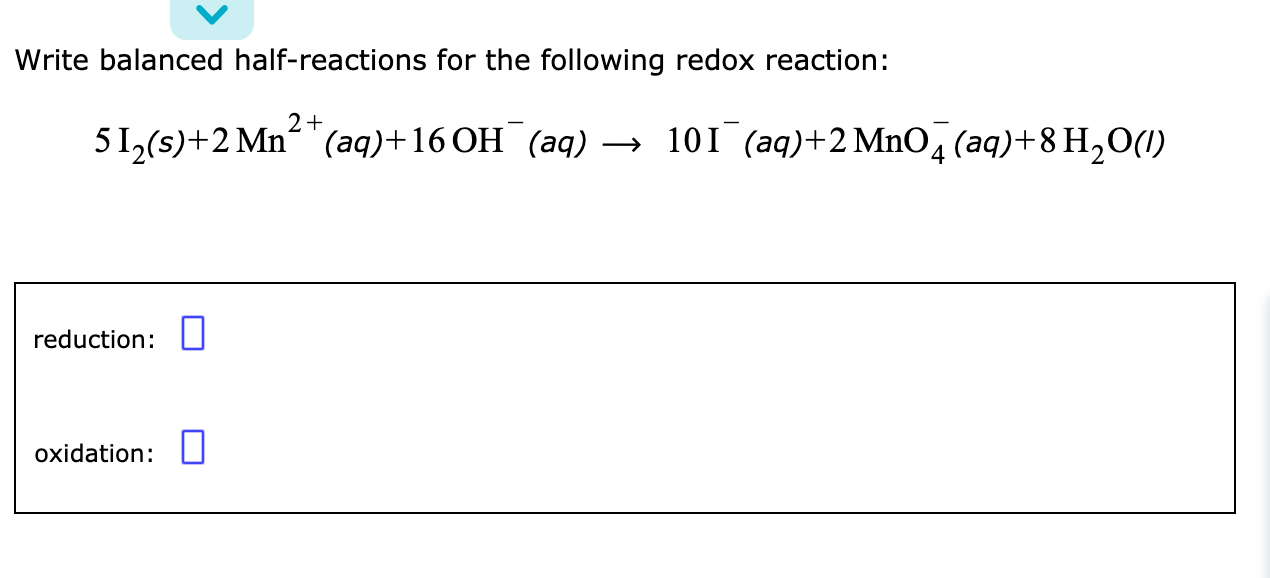Write balanced half-reactions for the following redox reaction: 512(3)+2 Mn²+ (aq)+160H (aq) → 101 (aq)+2 MnO2 (aq)+8 H20(1) reduction: oxidation:

• ### Write balanced half-reactions for the following redox reaction: Cr20, (aq) + 14 H + (aq)+3C2o;(a)-2Cr3 +(aq)...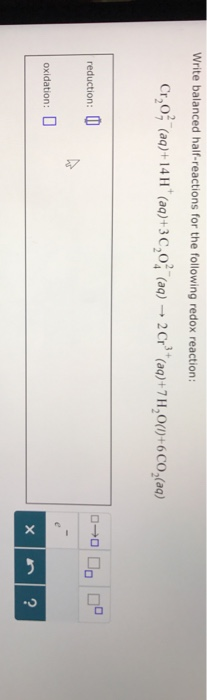Write balanced half-reactions for the following redox reaction: Cr20, (aq) + 14 H + (aq)+3C2o;(a)-2Cr3 +(aq) +7 H20(,)+6CO2(aq) reduction oxidation: 2

• ### (1) Identify each of the following half-reactions as either an oxidation half-reaction or a reduction half-...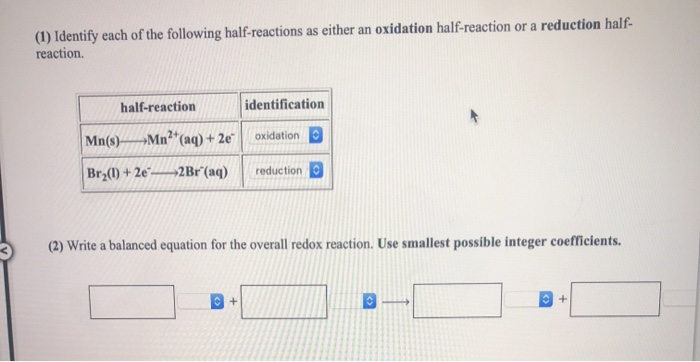(1) Identify each of the following half-reactions as either an oxidation half-reaction or a reduction half- reaction. identification half-reaction Mn(s) >Mn2(aq) + 2e Br2(1) +20_ 2Br" (aq) oxidation reduction (2) Write a balanced equation for the overall redox reaction. Use smallest possible integer coefficients.

• ### Balance the following oxidation-reduction reactions using the half-reaction method. 1. HCOOH (aq) + MnO.. (aq) → CO2 (g) + Mn2. Acidie solution Identify the reduction half Identify the oxidation...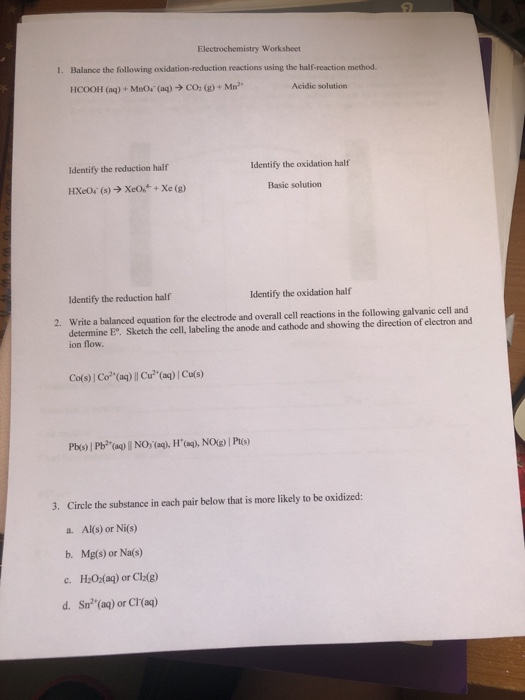Balance the following oxidation-reduction reactions using the half-reaction method. 1. HCOOH (aq) + MnO.. (aq) → CO2 (g) + Mn2. Acidie solution Identify the reduction half Identify the oxidation half Basic solution Identify the reduction half Identify the oxidation half Write a balanced equation for the electrode and overall cell reactions in the following galvanic cell and determine E°. Sketch the cell, labeling the anode and cathode and showing the direction of electron and ion flow. 2. 3. Circle the...

• ### (1) Identify each of the following half-reactions as either an oxidation half-reaction or a reduction half...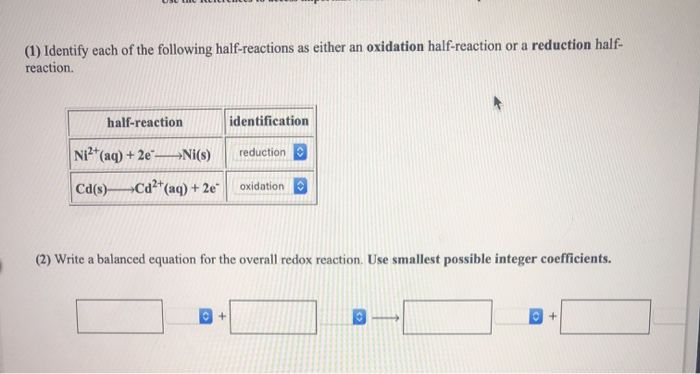(1) Identify each of the following half-reactions as either an oxidation half-reaction or a reduction half reaction. identification half-reaction Ni (aq)+2e reduction Ni(s) Cd2 (aq) +2e oxidation Cd(s)- (2) Write a balanced equation for the overall redox reaction. Use smallest possible integer coefficients. + +

Free Homework App